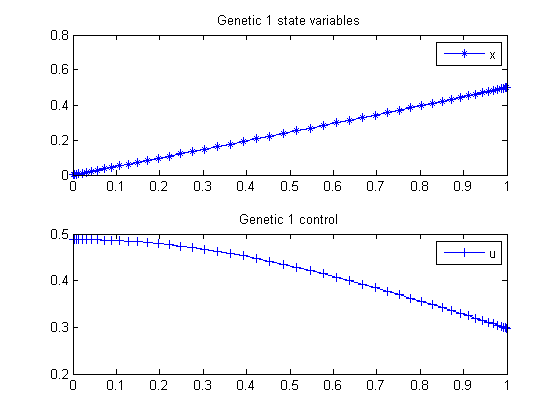40  Genetic 1

PROCEEDINGS OF WORLD ACADEMY OF SCIENCE, ENGINEERING AND TECHNOLOGY VOLUME 21 JANUARY 2007 ISSN 1307-6884

Optimal Control Problem, Quasi-Assignment Problem and Genetic Algorithm Omid S. Fard and Akbar H. Borzabadi

See paper for failure of GA toolbox algorithm.

Example 1

40.1  Problem Formulation

Find u over t in [0; 1 ] to minimize

J =
 1 0
u2 dt

subject to:

 dx dt
= x2 + u

The initial condition are:

 x(0) = 0
 x(1) = 0.5

Reference: 

40.2  Problem setup

toms t
p = tomPhase('p', t, 0, 1, 50);
setPhase(p);

tomStates x
tomControls u

% Initial guess
x0 = {icollocate(x == 0.5*t); collocate(u == 0)};

% Boundary constraints
cbnd = {initial({x == 0}); final({x == 0.5})};

% ODEs and path constraints
ceq = collocate({dot(x) == x.^2+u});

% Objective
objective = integrate(u.^2);

40.3  Solve the problem

options = struct;
options.name = 'Genetic 1';
solution = ezsolve(objective, {cbnd, ceq}, x0, options);
t = subs(collocate(t),solution);
x = subs(collocate(x),solution);
u = subs(collocate(u),solution);
Problem type appears to be: qpcon
Starting numeric solver
===== * * * =================================================================== * * *
TOMLAB - Tomlab Optimization Inc. Development license  999001. Valid to 2011-02-05
=====================================================================================
Problem: ---  1: Genetic 1                      f_k       0.178900993395128240
sum(|constr|)      0.000000000698273828
f(x_k) + sum(|constr|)      0.178900994093402070
f(x_0)      0.000000000000000000

Solver: snopt.  EXIT=0.  INFORM=1.
SNOPT 7.2-5 NLP code
Optimality conditions satisfied

FuncEv    1 ConstrEv   23 ConJacEv   23 Iter   22 MinorIter   71
CPU time: 0.093750 sec. Elapsed time: 0.094000 sec.

40.4  Plot result

subplot(2,1,1)
plot(t,x,'*-');
legend('x');
title('Genetic 1 state variables');

subplot(2,1,2)
plot(t,u,'+-');
legend('u');
title('Genetic 1 control');